## Example Questions

1 2 4 Next →

### Example Question #8 : How To Multiply Binomials With The Distributive Property

Which expression is equal to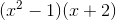?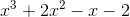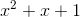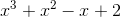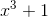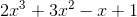Explanation:

In this problem, we are to multiply the two binomials using the FOIL method. This method stands for the order in which you multiply the variables. F stands for the first term of each binomial. O stands for the outside terms, meaning the first term of the first binomial and the last term of the second. I stands for the inside terms, meaning the second term of the first binomial and the first term of the second. L stands for the last term of each binomial. Once you do this, you simply add each part together to recieve your polynomial answer. Here is how our problem is solved: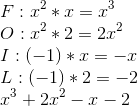1 2 4 Next →

### All ACT Math Resources